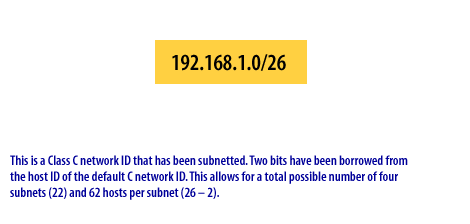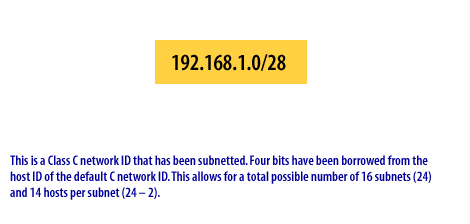DNS Lookup  «Prev  Next»
 Lesson 7 IP address subnet requirements Objective Determine the number of hosts per subnet and number of subnets

An IP-routed network design requires that you examine the interrelationship between the number of hosts per subnet and the number of subnets. An IP-switched network design evaluates only the number of WAN connections.
Your network design must optimize the number of subnets and the number of hosts per subnet. In designing an IP network and selecting the masks required to permit routing, you may be limited by:
1. The number of physical subnets that exist.
2. The number of logical subnets that you can create.
3. The host population on both physical and logical subnets.
A good subnet mask design does not restrict expected growth in either the number of subnets or the number of hosts per subnet. You need to adjust the subnet mask to provide for expected growth for both the number of hosts on each subnet and the number of subnets overall.

## Limits on Number of hosts per subnet

Consider the following in determining the number of hosts per subnet:
1. Network design specifications: Create your network design specifications to meet required performance goals. This requires analyzing the bandwidth utilization, broadcast domain size, routing configuration, distance vector delays, and application data flow requirements.
2. Router performance: Evaluate the number of hosts supported by any new or existing routers. To determine the maximum number of hosts supported per subnet, divide the total number of hosts on any LAN by the number of subnets supported by the router(s). If this number exceeds the host capacity of a subnet (as determined by your subnetting scheme) or limits performance (because of a large collision domain), then redesign the network to increase the number of subnets.
It may be necessary to test how many hosts you can support on a subnet before client performance is affected. These tests must use production applications.

1. Future growth: Examine the subnet mask to determine if the number of hosts per subnet provides for current needs, performance expectations, and future growth.

### Limits on the Number of Subnets

Use the following options to determine the validity of a subnet mask in an IP-routed network:
1. Subnet for each WAN connection: Examine the network design for any remote connectivity. To support routing, each remote connection requires a subnet.
2. Overloaded segments: Evaluate the number of hosts supported by any new or existing router. To determine the minimum number of routers required at any single location, divide the total number of hosts by the number of hosts optimally supported by the router.
3. Future growth: Examine the subnet mask to determine if the number of subnets and the number of hosts per subnet provide for growth. If possible, design for an excess of subnets, because router technology usually limits the number of hosts per subnet.

The following Slide Show provides further explanation and examples of IP address subnet requirements.1) This is a Class C network ID that has not been subnetted. You can represent this in binary as 11000000.10101000.00000001.00000000 11111111.11111111.11111111.00000000 Each bit in the IP address has a corresponding bit in the subnet mask. Those bits that correspond to the '1' in the subnet mask are considered part of the network ID.2) This is a Class C network ID that has been subnetted. Two bits have been borrowed from the host ID of the default C network ID. This allows for a total possible number of four subnets (22) and 62 hosts per subnet (26-2).3) This is a Class C network ID that has been subnetted. Four bits have been borrowed from the host ID of the default C network ID. This allows for a total possible number of 16 subnets (24) and 14 hosts per subnet (24-2).